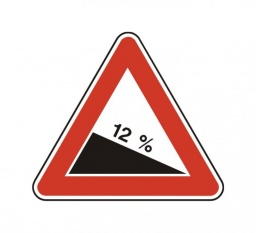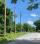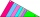The angle of a straight road is approximately 12 degrees. Determine the percentage of this road.

p =  21.3 %

### Step-by-step explanation:Did you find an error or inaccuracy? Feel free to write us. Thank you!Tips to related online calculators

#### You need to know the following knowledge to solve this word math problem:

We encourage you to watch this tutorial video on this math problem:

## Related math problems and questions:On a straight stretch of road is marked 12 percent drop. What angle makes the direction of the road with the horizontal plane?
• Right angled triangle 3Side b = 1.5, hypotenuse angle A = 70 degrees, Angle B = 20 degrees. Find its unknown sides length.
• Right triangleIt is given a right triangle angle alpha of 90 degrees beta angle of 55 degrees c = 10 cm use Pythagorean theorem to calculate sides a and bThe average climb of the road is given by ratio 1:15. By what angle road average climb?
• MastMast has 13 m long shadow on a slope rising from the mast foot in the direction of the shadow angle at angle 13.3°. Determine the height of the mast, if the sun above the horizon is at angle 45°12'.
• Right triangleA right triangle ABC is given, c is a hypotenuse. Find the length of the sides a, b, the angle beta if c = 5 and angle alfa = A = 35 degrees.
• Right triangle trigonometricsCalculate the size of the remaining sides and angles of a right triangle ABC if it is given: b = 10 cm; c = 20 cm; angle alpha = 60° and the angle beta = 30° (use the Pythagorean theorem and functions sine, cosine, tangent, cotangent)
• ClimbOn the road sign, which informs the climb is 8.7%. The car drive 5 km along this road. What is the height difference that the car went to?
• Right triangleCalculate the length of the remaining two sides and the angles in the rectangular triangle ABC if a = 10 cm, angle alpha = 18°40'.
• RightDetermine angles of the right triangle with the hypotenuse c and legs a, b, if: 3a +4b = 4.9c
• ClimbRoad has climbing 1:27. How big is a angle corresponds to this climbing?Between cities A and B is route 13 km long of stúpanie average 7‰. Calculate the height difference of cities A and B.Calculate the length of the side GN and diagonal QN of rectangle QGNH when given: |HN| = 25 cm and angle ∠ QGH = 28 degrees.What height difference overcome if we pass road 1 km long with a pitch21 per mille?On a straight road, three pillars are 6 m high at the same distance of 10 m. At what angle of view does Vlado see each pillar if it is 30 m from the first and his eyes are at 1.8 m high?In triangle ABC, right-angled at B. Sides/AB/=7cm, /BC/=5cm, /AC/=8.6cm. Find to two decimal places. A. Sine C B. Cosine C C. Tangent C.The point (3, 4) is on the terminal side of angle θ. cos θ = ...